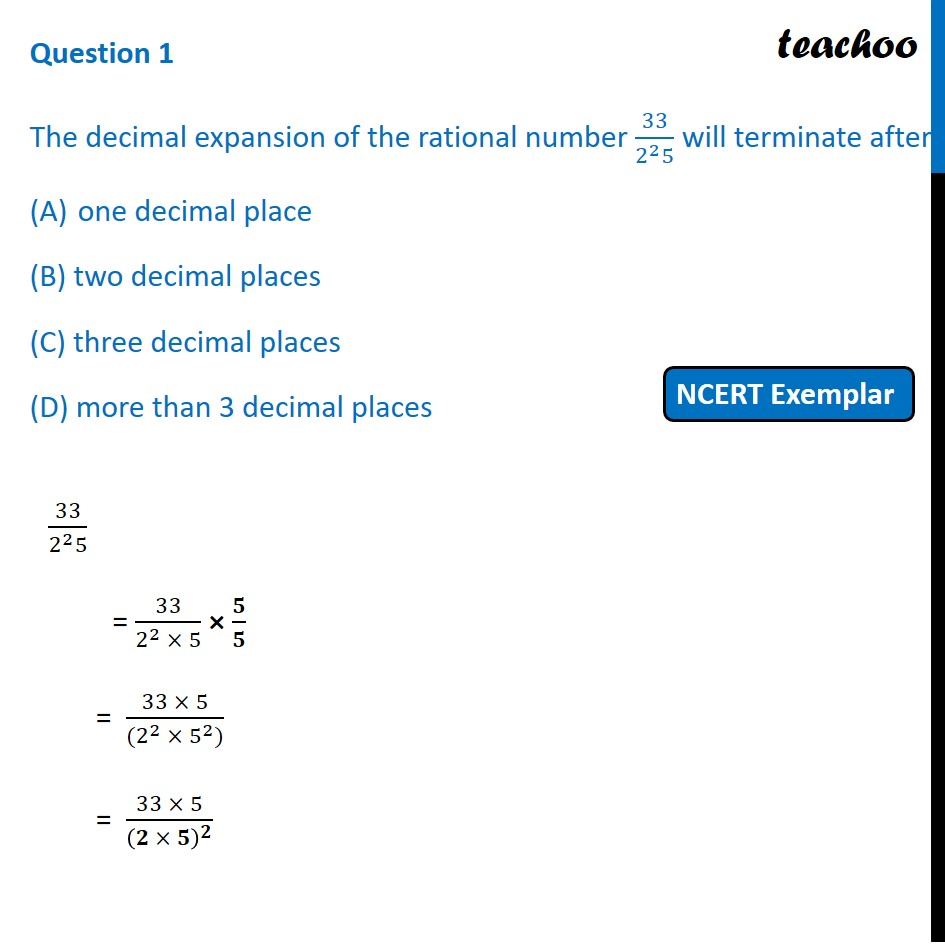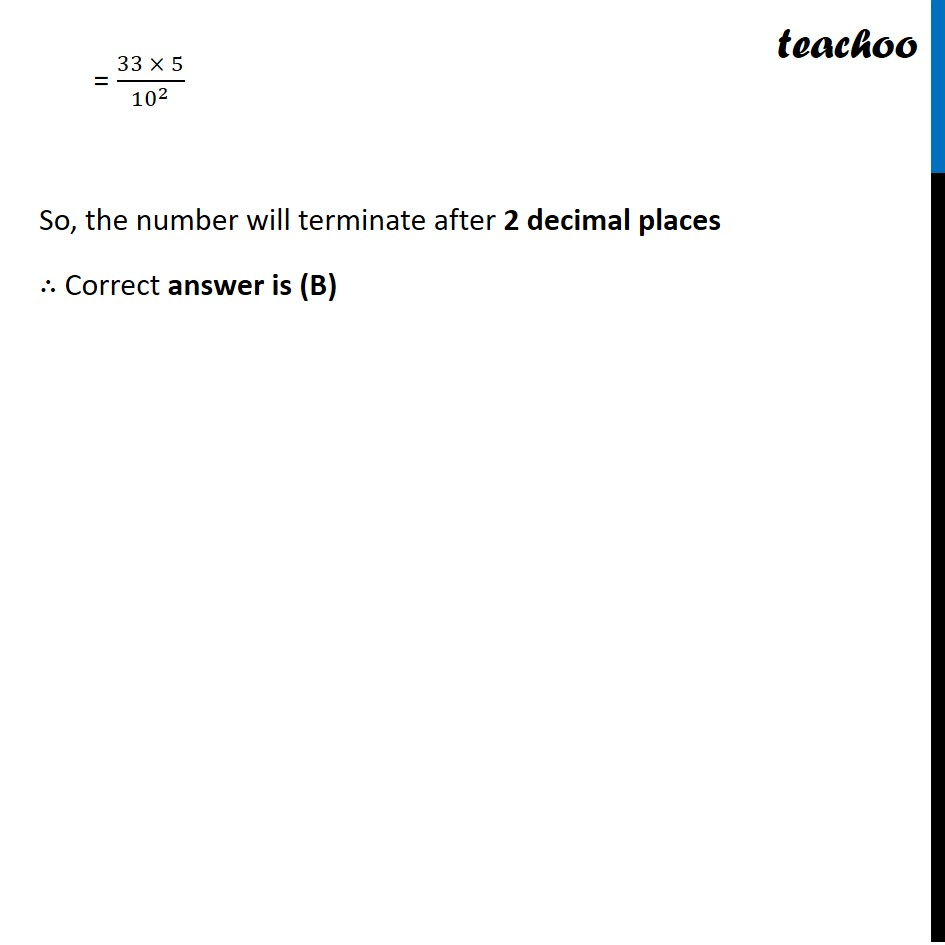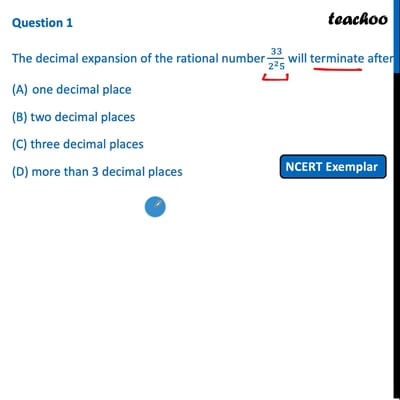MCQs from NCERT Exemplar

Chapter 1 Class 10 Real Numbers
Serial order wise

## (D) more than 3 decimal placesThis video is only available for Teachoo black users

Maths Crash Course - Live lectures + all videos + Real time Doubt solving!

### Transcript

Question 1 The decimal expansion of the rational number 33/(2^2 5) will terminate after one decimal place (B) two decimal places (C) three decimal places (D) more than 3 decimal places 33/(2^2 5) = 33/(2^2 × 5) × 𝟓/𝟓 = (33 × 5)/((2^2 × 5^2)) = (33 × 5)/(𝟐 × 𝟓)^𝟐 = (33 × 5)/〖10〗^2 So, the number will terminate after 2 decimal places ∴ Correct answer is (B)